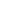Equals FunctionTest whether the two Atoms are the same.Syntax

 [C#] ```bool Equals(Atom other) override bool Equals(object test) bool Equals(Atom other, ComparisonType type)``` [Visual Basic] ```Function Equals(other As Atom) As Boolean Overrides Function Equals(test As Object) As Boolean Function Equals(other As Atom, type as ComparisonType) As Boolean```Params

 Name Description other The object to test against. type The type of comparison to perform. return Whether the objects are equal.Notes

 This method can be used to determine whether the specified object is equal to the current object. Objects are considered equal if they have the same content - so this method determines value equality rather than object equality. The function taking an object is required as part of the .NET framework, it performs exactly the same task as the function taking an Atom. The override taking a ComparisonType allows you to choose between different ways of evaluating equality. The enum is flags based so you can combine different options. Only if all options evaluate to true will true be returned. The ComparisonType provides the following options: None - Equal as long as the other atom is not null. Object - Equal if the atoms are object equal. Atom - Equal if the atoms are value equal.Example

 None.